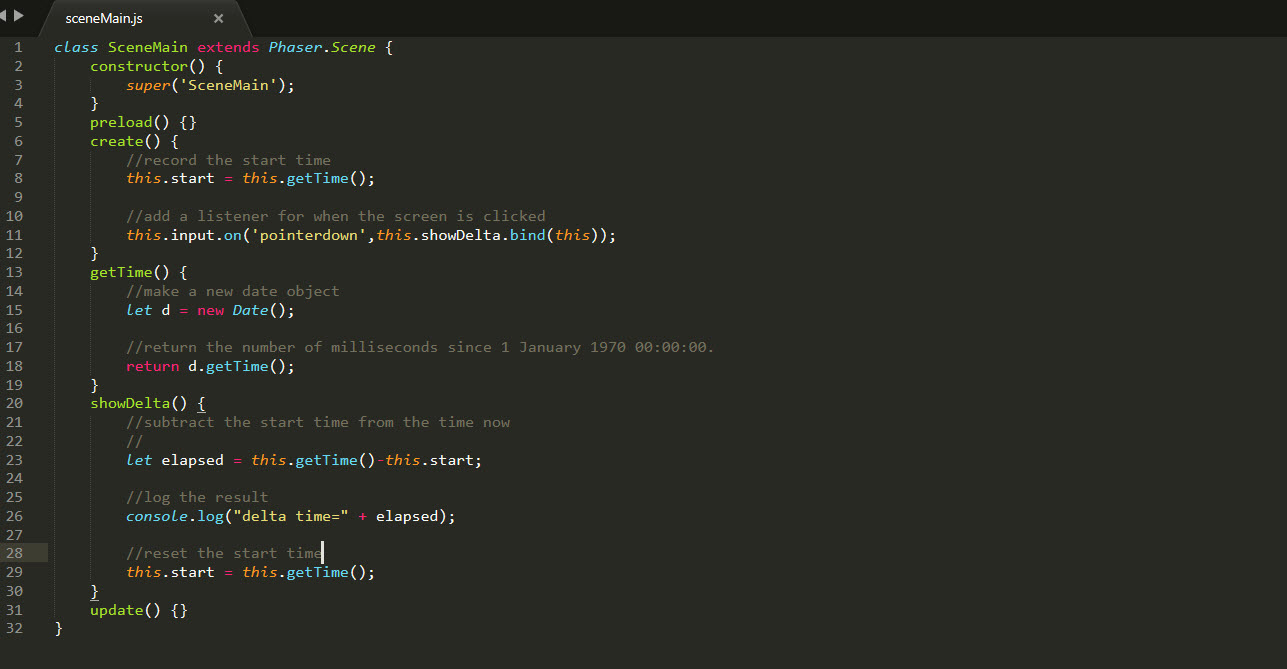# How to get Delta Time in Phaser 3

Recently I had a project that required me to use Unity and I liked how the deltaTime was built in. I’ve been using elapsed time in a lot of code from Flash to Phaser, but it occurred to me that developers that were moving between Unity and Phaser might be looking for a way to get the Delta or elapsed time between two different events. In this simple example, I use the “pointerdown” event to show time between clicks.

``````class SceneMain extends Phaser.Scene {
constructor() {
super('SceneMain');
}
create() {
//record the start time
this.start = this.getTime();

//add a listener for when the screen is clicked
this.input.on('pointerdown',this.showDelta.bind(this));
}
getTime() {
//make a new date object
let d = new Date();

//return the number of milliseconds since 1 January 1970 00:00:00.
return d.getTime();
}
showDelta() {
//subtract the start time from the time now
//
let elapsed = this.getTime()-this.start;

//log the result
console.log("delta time=" + elapsed);

//reset the start time
this.start = this.getTime();
}
update() {}
}``````